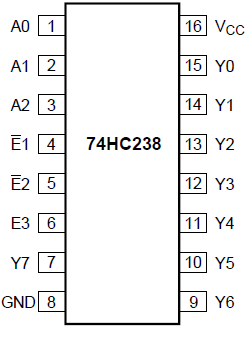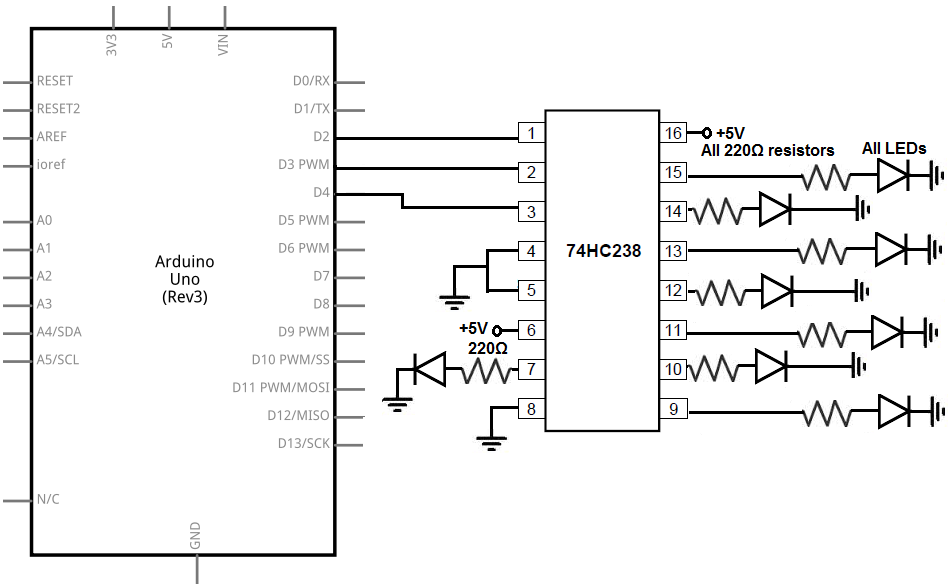﻿ How to Connect a 74HC238 3-to-8 Decoder/Demultiplexer to an Arduino ﻿# How to Connect a 74HC238 3-to-8 Decoder to an ArduinoIn this project, we will show how to connect an 74HC238 3-to-8 decoder/demultiplexer to an arduino micrcocontroller.

Using this decoder/demultiplexer, we can control 8 outputs with just 3 pins.

This is the reason decoder/demultiplexer chips are great additions to microcontrollers. With just 3 pins on the microcontroller being used, it adds 8 outputs. So it's a gain of +5 pins. So it functions as an output port expander. It can't be used as inputs so it doesn't have that dual capability of I/O ports, but if you only need pins to function as outputs, the 74HC238 and other decoder/demultiplexer chips is a great accessory tool to a microcontroller.

In this project, we will show how a 74HC238 decoder works and how it can be controlled with an arduino microcontroller.

Using 3 pins, called the address pins, we can create a total of 8 different outputs. This is because 23= 8.

Our output devices will be LEDs. Since there are 8 outputs on the 74HC238 chip, we will attach 8 LEDs to the chip. One output will have an LED along with a series-limiting resistor to limit excess current to the LED.

How the circuit works, in a nutshell, is there are 3 address pins, pins A0, A1, and A2. These address pins control which output pins turn on. So, again, with 3 pins, we can control 8 outputs.

The 3 pins can be in either 1 of 2 states. They can either be LOW (tied to GND) or HIGH (tied to VCC).

So if we have an address pin connected to ground, it has a value of 0. If an address pin is connected to +5V, it has a value of 1.

So if all address pins are connected to ground, this gives a value of 000. This equates to all the outputs (LEDs) being off.

If the address pins have a value of 001, this equates to the first LED being on.

If the address pins have a value of 010, this equates to the first 2 LEDs being on.

If the address pins have a value of 011, this equates to the first 3 LEDS being on.

If the address pins have a value of 100, this equates to the first 4 LEDs being on.

If the address pins have a value of 101, this equates to the first 5 LEDs being on.

If the address pins have a value of 110, this equates to the first 6 LEDs being on.

If the address pins have a value of 111, this equates to all 7 LEDs being being on.

And this how the address pins can affect all 8 outputs.

We will go into all the more details below.

But a 74HC238 is a very useful chip that has a lot of capabilities.

Components

• 74HC238 3-to-8 Decoder Chip
• 8 220Ω resistors
• 8 LEDs
• Arduino microcontroller

The 74HC238 3-to-8 decoder chip can be obtained for under \$1 on ebay.

It is a 8-pin chip.

The datasheet for this chip can be found at the following link: MCP23008 Datasheet.

The pinout of the 74HC238 is shown below.The 74HC238 can be powered with +5V. Therefore, we can connect VCC to We connect VSS to ground. This completes the powering that's necessary for the 74HC238.

The A0, A1, and A2 pins are the address pins. These are the pins which are used to control the outputs of the chip. It follows a binary-to-decimal code.

So if all address pins are HIGH, this equates to a value of 111, which in decimal is 7. So when all address pins are HIGH, all 7 LEDs will be on.

If the A0 is HIGH, A1 is LOW, and A2 is HIGH, this equates to a value of 101, which in decimal is 5. So when the address pins are 101, 5 LEDs will be on.

The address pins are kind of the center of control.

The enable pins are the pins that allow for outputs to be able to turn on when E1 and E2 are LOW and E3 is HIGH. without this combination, none of the output pins can turn on. In other words, every output will be LOW unless E1 and E2 are LOW and E3 is HIGH.

The rest of the pins, Y0 to Y7, are the output pins. To these pins, we connect an LED along with a series-limiting resistor to limit excess current to the LEDs, so they don't get blown out.

### 74HC238 3-to-8 Decoder/Demultiplexer Circuit with an Arduino Microcontroller

The 74HC238 3-to-8 decoder/demultiplexer circuit we will build with an arduino microcontroller is shown below.We will now explain the hardware connections.

First to connect power, we connect VCC to the +5V terminal of the arduino and the GND pin to the GND terminal of the arduino.

The first 3 pins of the microcontroller are A0, A1, and A2. We connect these pins to digital pins 2, 3, and 4. By writing these pins HIGH or LOW, we can control the LEDs at the outputs.

The next 3 pins are the enable pins, E1, E2, and E3. Since we always want output to be enabled, we permanently connect E1 and E2 to GND and connect E3 to +5V.

The rest of the pins, Y0 to Y7, are outputs. To each of these we connect an LED and a series-limiting resistor.

How the circuit works is the pushbuttons control the outputs. Without any pushbutton being pressed, all the LEDs are off. If we press down on the pushbuttons, the corresponding LEDs will turn on in a binary-to-decimal format.

If we push down on the first 2 pushbuttons attached to A0 and A1, this gives a value of 011 to the address registers. This is the equivalent in decimal of 3, so the first 3 LEDs turn on.

You have to know binary and decimal equivalents to really work with this well. But once you do, it's very simple and straightforward to work.

### Code

The code to turn on the LEDs one at a time from the first to the last LED with a 74HC238 is shown below.

//74HC238 Decode/Demultiplexer Circuit

const int AddressPin0= 2; //pin A0 connects to digital pin 2
const int AddressPin1= 3; //pin A1 connects to digital pin 3
const int AddressPin2= 4; //pin A2 connects to digital pin 4

void setup()
{
//sets all the address pins as outputs
}

void loop()
{
digitalWrite(AddressPin0,HIGH); //turns the first LED only
delay(1000); //waits 1 second

digitalWrite(AddressPin0,LOW); //turns the first 2 LEDs
delay(1000); //waits 1 second

digitalWrite(AddressPin0,HIGH); //turns on the first 3 LEDs
delay(1000); //waits 1 second

digitalWrite(AddressPin0,LOW); //turns on the first 4 LEDs
delay(1000); //waits 1 second

digitalWrite(AddressPin0,HIGH); //turns on the first 5 LEDs
delay(1000); //waits 1 second

digitalWrite(AddressPin0,LOW); //turns on the first 6 LEDs
delay(1000); //waits 1 second

digitalWrite(AddressPin0,HIGH); //turns on all 7 LEDs
delay(1000); //waits 1 second

delay(1000); //waits 1 second
}

The first block of code declares and initializes the three address pins, A0, A1, and A2 to their pin connections. So A0 is set to 2, A1 is set to 3, and A2 is set to 4.

After this, in our setup() function, we declare these address pins as outputs. This is because we are writing to them with the arduino, not reading from them. So they are declared outputs. Outputs are devices the arduino writes to. Inputs are devices the arduino reads from. So the 74HC238 address pins are outputs to the arduino.

After this, we have our loop function. This is the function that repeats over and over and over again until externally stopped. In this function, we first turn on 1 LED, then 2, then 3, then 4, 5, 6, then all 7 LEDs, and then shut all LEDs off, with one-second delays between each step. Then it repeats back from the beginning.

You can, of course, this circuit at any way you want to, for any type of output to turn on and turn on for any given set of time, or for an output to just always be on. It's easy to modify it with software.

And this is how a decoder chip works to give a microcontroller extra output ports and increased ease of controlling outputs.

Related Resources

How to Build a 74HC238 3-to-8 Decoder Circuit with Manual Pushbuttons